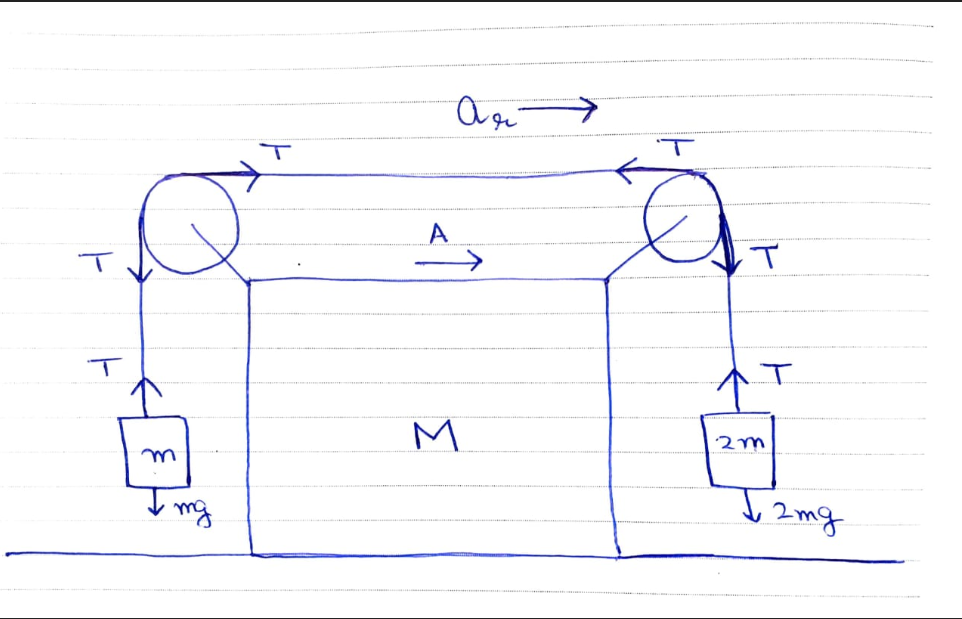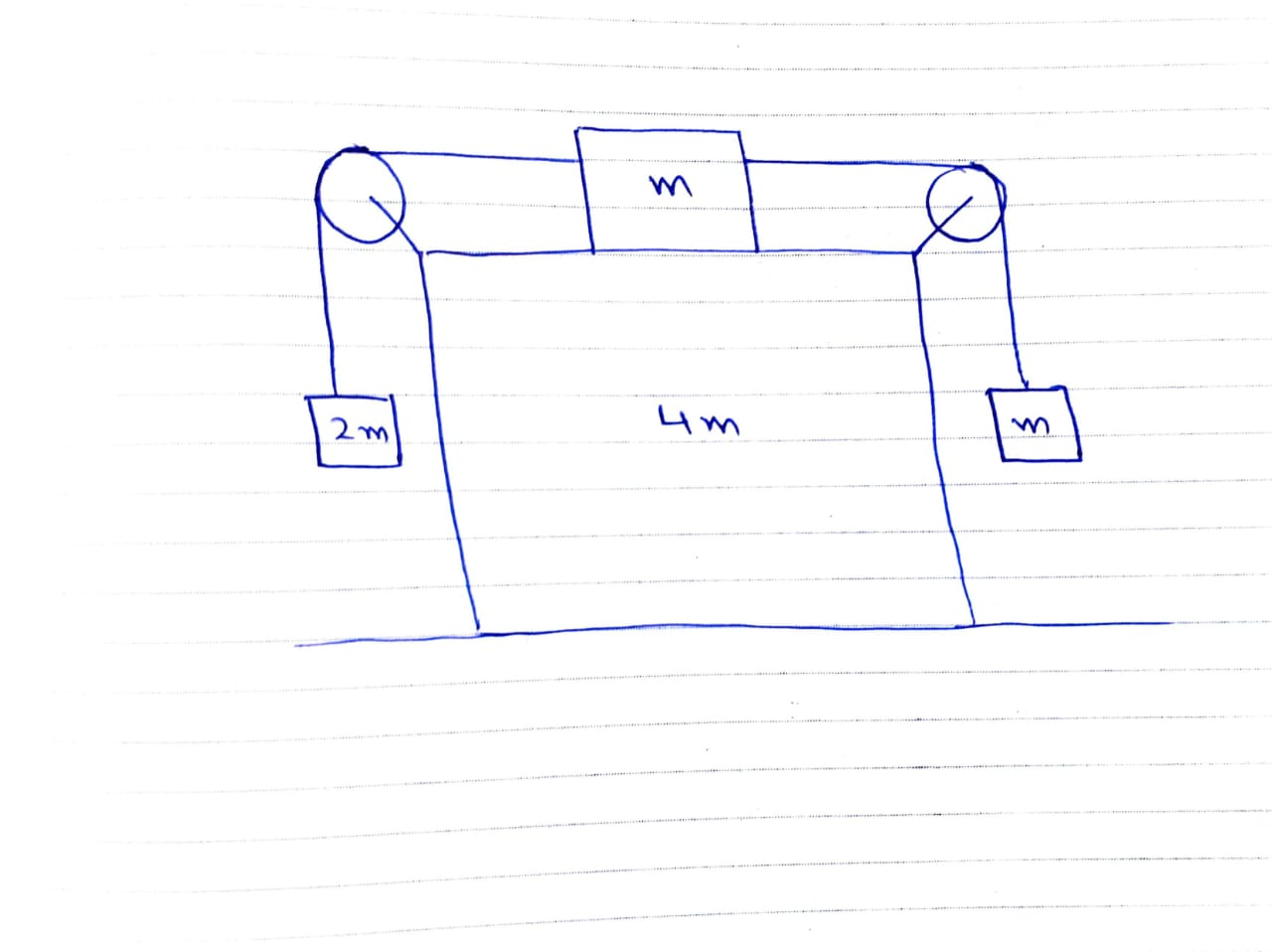# Finding accelerationWe have to find the acceleration of the blocks in the above picture considering all the surfaces to be smooth and tension is in string is uniform.

Since, in the horizontal direction, there is no force, that implies that the block of mass $M$ does not have any acceleration.

Therefore $A=0$.

Now, if the blocks of mass $2m$ and $m$ are moving with an acceleration $a_r$ relative to the bigger block (of mass $M$) .

Now, we can write the force equations for the smaller blocks, that is $2mg-T=2ma_r$ and $T-mg=ma_r$.

Solving this, we get $a_r=\dfrac{g}{3}$. However, the answer given to the above problem is $a_r=0$ as well, that is , whole system is at rest. Is my method wrong or the given answer is wrong?

Another similar problem that is bothering me is this:Now, here similar to the previous problem I have assumed that the bigger block of mass $4m$ moves towards right and the smaller blocks move with an acceleration $a_r$ relative to the bigger bloc towards left.

Again in horizontal direction, net force is $0$ so I wrote $4mA+m(A-a_r)=0 \implies a_r=5A$ but the given answer in this case is $A=\dfrac{g}{23}$ and $a_r=\dfrac{6g}{23}$. Now my very first equation becomes wrong due to this. Where is the fault?Note by Vilakshan Gupta
10 months ago

This discussion board is a place to discuss our Daily Challenges and the math and science related to those challenges. Explanations are more than just a solution — they should explain the steps and thinking strategies that you used to obtain the solution. Comments should further the discussion of math and science.

When posting on Brilliant:

• Use the emojis to react to an explanation, whether you're congratulating a job well done , or just really confused .
• Ask specific questions about the challenge or the steps in somebody's explanation. Well-posed questions can add a lot to the discussion, but posting "I don't understand!" doesn't help anyone.
• Try to contribute something new to the discussion, whether it is an extension, generalization or other idea related to the challenge.
• Stay on topic — we're all here to learn more about math and science, not to hear about your favorite get-rich-quick scheme or current world events.

MarkdownAppears as
*italics* or _italics_ italics
**bold** or __bold__ bold
- bulleted- list
• bulleted
• list
1. numbered2. list
1. numbered
2. list
Note: you must add a full line of space before and after lists for them to show up correctly
paragraph 1paragraph 2

paragraph 1

paragraph 2

[example link](https://brilliant.org)example link
> This is a quote
This is a quote
    # I indented these lines
# 4 spaces, and now they show
# up as a code block.

print "hello world"
# I indented these lines
# 4 spaces, and now they show
# up as a code block.

print "hello world"
MathAppears as
Remember to wrap math in $$ ... $$ or $ ... $ to ensure proper formatting.
2 \times 3 $2 \times 3$
2^{34} $2^{34}$
a_{i-1} $a_{i-1}$
\frac{2}{3} $\frac{2}{3}$
\sqrt{2} $\sqrt{2}$
\sum_{i=1}^3 $\sum_{i=1}^3$
\sin \theta $\sin \theta$
\boxed{123} $\boxed{123}$

## Comments

Sort by:

Top Newest

Acceleration is $\displaystyle a = \frac{g}{3}$ as you claim. Maybe both blocks have equal mass $m$ or the answer given is wrong. :)

- 10 months ago

Log in to reply

No, the blocks aren't of equal mass. I have one more similar problem, and again my answer does not match with the given answer. Give it a try too, I will upload it too.

- 10 months ago

Log in to reply

Ok, I'll give it a try. Do mention me there so that I'll get notified. :)

- 10 months ago

Log in to reply

Wait, are the blocks being moved to right by a force in the question?

- 10 months ago

Log in to reply

No. They are not. The system is released from rest. I have assumed the accelerations.

- 10 months ago

Log in to reply

I have uploaded it.

- 10 months ago

Log in to reply

×

Problem Loading...

Note Loading...

Set Loading...